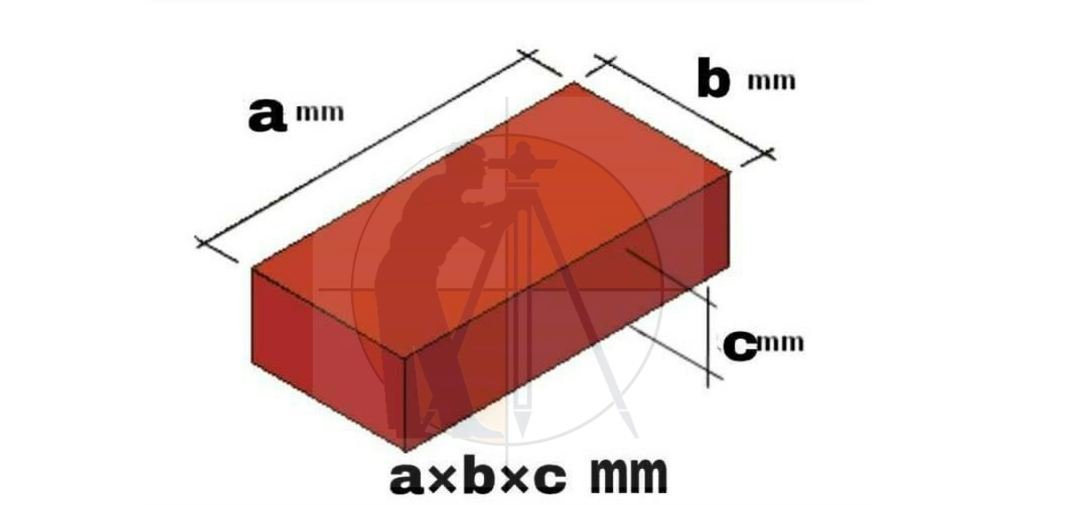Breaking News
Home / Civil Engineering / Basic Estimation And Quantity Of Materials For Brick Wall, U.C.R. Masonry Plastering And Tiles

# Basic Estimation And Quantity Of Materials For Brick Wall, U.C.R. Masonry Plastering And Tiles

### Basic Estimation And Quantity Of Materials For Brick Wall, U.C.R. Masonry Plastering And TilesCalculate the quantity of material for load-bearing wall with length 6 m thickness 40 cm and height 3.5 m preparation of mortar (1:6)

Volume of wall = base quantity
= b x d x t = l x h x t
= 6 x 3.5 x 0.4
= 8.4 m3

Standard diamension of brick with mortar = 0.2 x 0.1 x 0.1 = 0.002 m3

Number of bricks = 8.4 / 0.002 = 42.00 nos.

Actual volume of one brick = 0.19 x 0.9 x 0.9 = 1.539 x 10-3

Total volume of bricks = 4200 x 1.539 x 10-3 = 6.46 m3

Volume of mortar = 8.4 – 6.46 = 1.94

Actual volume of water required will 25 % more due to losses
= 1.94 + 25 % = 2.42 m3

Quantity of cement required
= 2.42 / 7 = 0.34 m3

1 m3 = 30 bag
0.35 x 30 = 10.5 bags say 11 bags

Quantity of sand required
= 0.35 x 6 = 2.1 m3
1 truck = 5.87 m3
Therefore 0.08 m3 = 0.35 trucks

Sr. no.
Item
Quantity
Rate
Total
1
Bricks
4200 Nos.
750/100 nos.
3150
2
Sand
1 ½ Brick
2000/truck
1000
3
cement
11 bag
110/bag
1210

5,360 Rs.

### Civil Site Engineer Must Know +18 Civil Engineer Tips And Trick

Partition wall with bricks

Find the estimation of material required for a building partition wall with brickwork second class, cement mortar 1:6 and base quantity 10 m3

Total volume = base quantity = 10 m3

Volume of one brick with mortar
= 0.2 x 0.1 x 0.1 = 0.002 m3

No. of bricks required = 10 / 0.002 = 5000 nos.

Actual volume of one brick
= 0.10 x 0.9 x 0.9
= 1.539 x 10-3 x 5000
= 7.69 m3 say 7.7 m3

Actual volume of mortar = 10 – 7.7 = 2.3 m3

Considering 25 % more for losses.

Actual volume of mortar required
= 2.3 + 25 % 2.3 = 2.87 m3

Quantity of cement required
=2.87 / 7 = 0.41 m3
= 0.41 x 30 = 12.3 bags say 13 bags

Quantity of sand required
= 0.41 x 6 = 2.46 m3
1 truck = 5.87 m3
2.46 m3 = ½ truck

Sr. no.
Items
Quantity
Rate
Total
1
Bricks
5000
750/1000
3750
2
Sand
½ truck
2000/truck
1000
3
Cement
1/13 bag
110/bag
1430

6,180 Rs.

### How To Measure Quantity Of Sand In Truck & HAIWA

Estimate for the material required for load-bearing wall of U.C.R. masonry consider base quantity 10m3 and proportion of mortar (1:6)

Quantity of stones required = 125 % of base
Quantity = 12.5 m3

Quantity of mortar required
Considering all stones.
42 % of base quantity = 4.2 m3

Quantity of cement required
= 4.2/7 = 0.6 m3
1m3 = 30 bags
0.6m3 = 18 bags

Quantity of sand required
= 0.6 x 6 = 3.6 m3
1 truck = 5.87 m3
Therefore 3.6 m3 = 0.6 trucks = 1 truck

Sr. no.
Items
Quantity
Rate
Total
1
Stone
13 m3
275/m3
3575
2
Sand
1 truck
2000/truck
2000
3
Cement
18 bags
110/bag
1980

7,555 Rs.

Estimate the quantity of material required for plastering the area 100 m2. Mix proportion used 1:5. The thickness of plaster is 12 mm.

Volume of mortars = 100 x 12 / 10 x 100 = 1.2 m3

Considering 25 % more due to losses
= 1.2 + 2.5 % . 12 = 1.5 m3

Quantity of cement required
= 1.5 / 6
=0.25 m3
0.25 m3 = 0.25 x 30
7.5 bags = 8 bags

Quantity of sand = 0.25 x 5 = 1.25 m3
1 truck = 5.87 m3
1.25 m3 = 0.21 truck
= ½ truck

Sr. no.
Items
Quantity
Rate
Total
1
Cement
8 bags
110/bag
880
2
Sand
½ truck
2000/truck
1000

1,880 Rs.

### How to Calculate Quantities Cement, Sand, Aggregate and water in 1 m3 Concrete Work

Calculate the estimate for mosaic tiles flooring over an area of 100 m2 with 20 mm thick lime.
Size of tile = 20 cm x 20 cm
Proportion of mortar = (1:2:4)

Base quantity = area = 100 m2
Size of tile = 20 cm x 20 cm
The thickness of lime base = 20 mm

Area of one tile = 20 cm x 20 cm
= 400 cm2 = 0.004 m2

Total no. of tiles required = 100 /0.04 = 25 00 nos.

Volume of lime base = 100 x (20 / 10 x 100) = 2 m3

Considering 25 % more due to looses = 2 + 25 % .2 = 2.5 m3

Quantity of cement required = 2.5 / 7 = 0.35 m3
1 m3 = 30 bags
0.35 x 30 = 10.5 bags say 11 bags

Consider 6 bags of cement for 100 m2 surface for slurry
= total quantity = 10.5 +6 = 16.5 say 17 bags

Quantity of lime required = 0.35 x 2 = 0.7 m3
1 m3 = 30 bags
7 m3 = 21 bags

Quantity of sand required = 0.35 x 4 = 1.4 m3 = ½ truck

Sr.no.
Items
Quantity
Rate
Total
1
Tiles
2500
750/brass
13020
2
Cement
17 bags
110/bag

### THANKS.